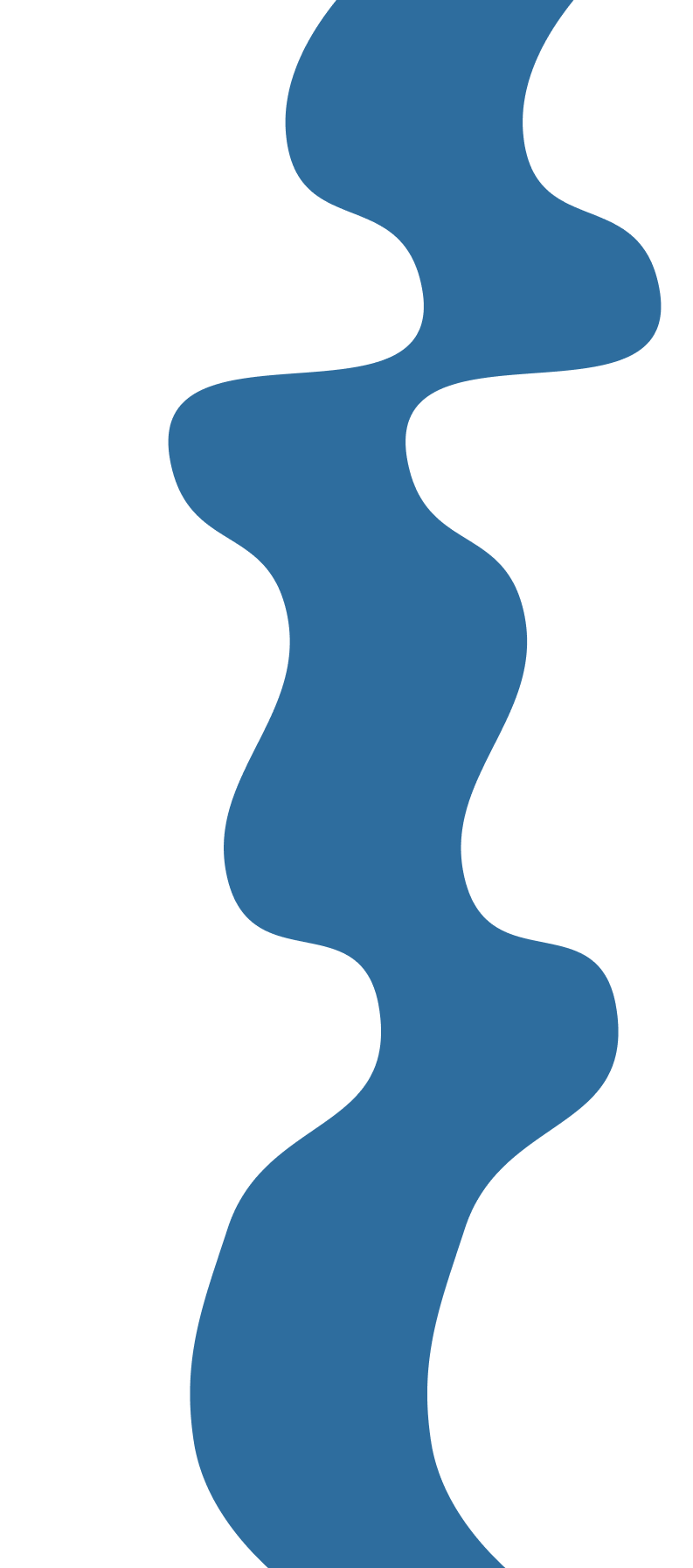Electrical EngineeringShayan Raofi

First thing to address is what is D.C. Steady State.  Basically, all that means is that the circuit has been active/running for a long time.  For power dissipating elements like resistors, this doesn’t mean much, but for energy storing elements such as inductors and capacitors it changes how they behave.

Capacitors become open circuits, which means that there is a break in the circuit, in D.C. steady state, while inductors become short circuits, which means they become a wire, in D.C. steady state.

A good way to remember this is to understand why this occurs.  Capacitors store charge in a electrostatic field.  Charge will continue to build up on the plates until the voltage gets to a maximum (usually equaling the voltage of the voltage source) and can no longer increase/change.  Given that the current through a capacitor is i = C(dv/dt), so capacitance times the derivative of the voltage across the capacitance, if the voltage is at a maximum, dv/dt = 0 since the voltage is no longer changing, so i= 0 and it’s an open circuit.  For inductors they store energy in a magnetic field, which is induced as current runs through their loops (an inductor, in its most basic form, is just a looped wire).  Its voltage is dependent on a changing current, v=L(di/dt), where L is the inductance and di/dt is the derivative of the current.  At DC steady state, the current no longer changes (it reaches a maximum through an inductor), so di/dt = 0 so the voltage is 0.

So to solve DC steady state problems all we have to do is change the capacitors into open circuits and the inductors into wires.  For problem 1, the steady state circuit would be:

The open circuit branch has no current running through it, so we can essentially pretend it’s not there:

Since we are just finding the current through one of two branches in parallel, we can get the current through the 2 ohm resistor by just using the current divider law, which states:

So:

NOTE: You can ignore the first 6 ohm resistor (the one next to the 12 amp current source) for the sake of using the current divider formula since the current through it is set by the current source, so the total current entering the two branches is still 12 amps (the current divider law is just concerned with how a total current is split between multiple branches).

Problem 2 is a bit trickier.  We start the same way, by setting the inductors to wires:

But we quickly run into a problem.  Both are short circuits, which normally means they divert all current flowing through adjacent/parallel branches through them (as current flows down the path of least resistance, and the resistance of a  wire is 0), but since there’s two of them, we have to determine which one gets more of the diverted current.

To do that, we just have to say that the currents that are flowing through the inductors are changing with time as they reach DC steady state, eventually reaching their maximum/DC steady state values. We can then find what they are right as they hit DC steady state.  That’s the theory anyway, in practice it's much easier than it sounds.  We essentially just have to do a current divider using the inductances instead of resistances.

So the first step like any current divider problem is to find the total current going into the two branches.  For this problem its just -4 A (or 4 amps pointing downwards).  This is because the two current sources, the 4 A and -8 A, are in parallel, so you can just add them.

We can also remove the two resistors, as the inductors are technically short circuits, so all -4 A will go through the inductors rather than the resistors.  With all that the circuits is simplified to:

We then apply current divider to find i1, then use that i1 + i2 = -4, since the total current just splits into the two branches, to find i2.

Problem 3 is much like problem 2 except with capacitors.  We set the circuit to DC steady state get two open circuits where the capacitors were:

This doesn’t tell us much about v1 and v2 except that they add up to 16 V since they are in parallel with the voltage source.  To actually find them, we do what we did for the inductors: we assume the voltages are changing in time before they reach their maximum/DC state, and get the voltage right as they hit DC steady state.  To do this in practice we use the voltage divider which states:

We just use the capacitances instead of the resistances for this case.Shayan Raofi## Schedule a demo

This won’t be sitting through a boring slideshow - we like to ask questions, learn about your workflows and pain points, and get creative with proposing meaningful solutions.